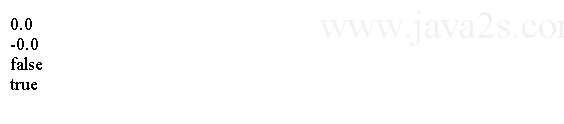# Java Tutorial - Java Floating Point

## float type

`float` type represents single-precision numbers.

`float` type variables are useful when you need a fractional component. Here are some example float variable declarations:

`float high, low;`

## Java float Value, size and Literals

float is 32-bit width and its range is from `1.4e-045` to `3.4e+038` approximately.

Floating-point literals in Java default to double precision. To specify a float literal, you must append an `F` or `f` to the constant.

The following code shows how to declare float literals.

```public class Main {
public static void main(String args[]) {
float d =  3.14159F;
System.out.print(d);//3.14159
}
}
```

The code above generates the following result.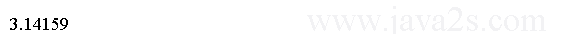## Java double type

Java double type represents double-precision numbers.

double is 64-bit width and its range is from 4.9e-324 to 1.8e+308 approximately.

Here is a program that uses double variables to compute the area of a circle:

```public class Main {
public static void main(String args[]) {
double pi, r, a;
// ww  w. j a  v  a 2 s  . co  m
r = 10.8888; // radius of circle
pi = 3.1415926; // pi, approximately
a = pi * r * r;

System.out.println("Area of circle is " + a);
}
}
```

The output: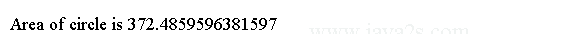## Example

double type numbers have decimal values with a fractional component. They can be expressed in either standard or scientific notation. Standard notation consists of a whole number component followed by a decimal point followed by a fractional component. For example, `2.0`, `3.14159`, and `0.6667`.

```public class Main {
public static void main(String args[]) {
double d =  3.14159;
System.out.print(d);//3.14159
}
}
```

The code above generates the following result.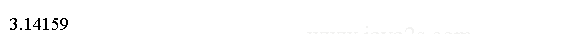## Example 2

You can explicitly specify a double literal by appending a D or d.

```public class Main {
public static void main(String args[]) {
double d =  3.14159D;
System.out.print(d);//3.14159
}
}
```

The code above generates the following result.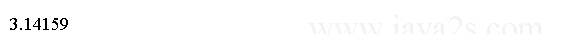## Scientific notation

Scientific notation uses a standard-notation, floating-point number plus a suffix that specifies a power of `10` by which the number is to be multiplied. The exponent is indicated by an E or e followed by a decimal number, which can be positive or negative. For example, `6.02E23`, `314159E-05`, and `4e+100`.

```public class Main {
public static void main(String[] argv) {
double d1 = 6.022E23;
double d2 = 314159E-05;
double d3 = 2e+100;
/*from   ww w.j a  v a  2s .  c om*/
System.out.println("d1 is " + d1);
System.out.println("d2 is " + d2);
System.out.println("d3 is " + d3);
}

}
```

The output generated by this program is shown here: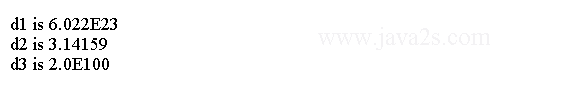## double value constant

Java's floating-point calculations are capable of returning `+infinity`, `-infinity`, `+0.0`, `-0.0`, and `NaN`

dividing a positive number by `0.0` returns `+infinity`. For example, `System.out.println(1.0/0.0);` outputs Infinity.

```public class Main{
public static void main(String[] args) {
System.out.println(1.0/0.0);
}

}
```

The code above generates the following result.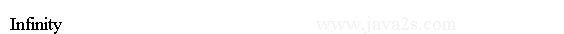## double Infinity

Dividing a negative number by `0.0` outputs `-infinity`. For example, `System.out.println(-1.0/0.0);` outputs `-Infinity`.

```public class Main{
public static void main(String[] args) {
System.out.println(-1.0/0.0);
}

}
```

Output: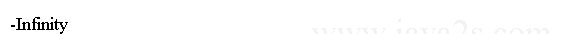## double NaN

Dividing `0.0` by `0.0` returns `NaN`. square root of a negative number is `NaN`. For example, `System.out.println(0.0/0.0)` and `System.out.println(Math.sqrt(-1.0))` output NaN.

Dividing a positive number by `+infinity` outputs `+0.0`. For example, `System.out.println(1.0/(1.0/0.0));` outputs `+0.0`.

Dividing a negative number by `+infinity` outputs `-0.0`. For example, `System.out.println(-1.0/(1.0/0.0));` outputs `-0.0`.

```public class Main {
public static void main(String[] args) {
Double d1 = new Double(+0.0);
System.out.println(d1.doubleValue());
/*from w ww.  jav  a2  s  . c  o m*/
Double d2 = new Double(-0.0);
System.out.println(d2.doubleValue());
System.out.println(d1.equals(d2));
System.out.println(+0.0 == -0.0);

}
}
```

The code above generates the following result.